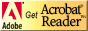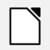Page last updated Friday May 12, 2017

I developed this calculator to do basic statistical analysis of a set of repeated measurements; specifically, measurements with uncertainties. (Note the field where you can enter the precision measure or the measuring instrument.)

Here's an OGG video about how to use the calculator.

You can fill in any of the cells to calculate statistics; they needn't be continuous or have a specific start or end cell number.

## Statistics Calculator

 N1 N6 N2 N7 N3 N8 N4 N9 N5 N10 Number of points Median Maximum Minimum Average Deviation (δ) Standard Deviation (σ) Standard Deviation of the Mean (α) Precision Measure of Instrument Average Uncertainty in Average Optimum Number of Measurements

## Operation:

Type in up to ten values in cells labelled N1 to N10. The values can be in any order, and cells can be left blank. Then press the "calculate" button to generate the statistics. For 2 or more data points, all statistics can be produced. you can change values individually or clear the whole set at once with the "clear" button provided.
If a precision measure is given, the uncertainty in the average is the larger of the precision measure and the standard deviation of the mean.
If a precision measure is given, the optimum number of measurements is the number for which the the precision measure and the standard deviation of the mean should be equal.
Note: The standard deviation calculated is the sample standard deviation, not the population standard deviation.

## ResourcesIf you need to update a browser, you might try Firefox which is
• free
• open source
• available for several platformsSince this page uses cascading style sheets for its layout, it will look best with a browser which supports the specifications as fully as possible.

If you are looking for an office package, with a word processor, spreadsheet, etc., you might try LibreOffice which is
• free
• open source
• available for several platformsGo to the main page for the Department of Physics and Computer Science.

Wilfrid Laurier University